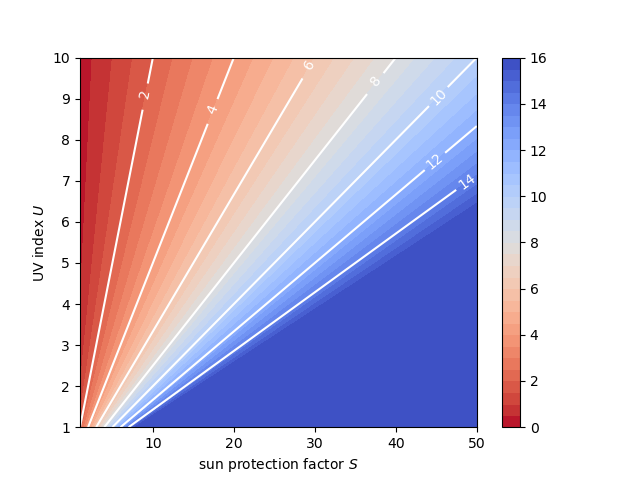## Sun Protection Factor to the Extreme

### How does the sun protection factor mean? Some calculations on the duration of protection with sunscreen.

It is well known that the sun protection factor SPF does not describe how much longer you can stay in the sun but rather how much of the damaging UV radiation is blocked. To get proper protection from UV radiation, you have to reapply sunscreen multiple times a day and still avoid staying in direct sunlight for extended periods of time. In this short post, I want to assume an idealized world, in which sunscreen simply extends the time you can stay in the sun without getting burnt by the SPF.

(Disclaimer: This is not medical advice!)

The time you can stay in the sun without getting burnt strongly depends on the UV index $U$, which is a dimensionless quantity proportional to the intensity of the damaging UV radiation. Many weather services provide this information in their weather forecasts, it is a great way of knowing when you can skip sunscreen and when you definitely need it.

The time $T$ skin can – on average – naturally resist getting burnt for radiation with UV index $U=8$ depends on the Fitzpatrick skin type:

skin type protection time without sunscreen $T$ emoji
1 10 min 👦🏻
2 15 min 🧑🏻
3 20 min 👩🏼
4 30 min 👨🏽
5 90 min 🧒🏾
6 120 min 👧🏿

Assume, that the sun protection factor SPF $S$ simply extends the time as a factor at $U=8$: $$T_8 = S T$$

Since the time until you get burnt is anti-proportional to the amount of radiation you are exposed to, we get for other UV indices $U$: $$T_U = \frac{8}{U} T_8 = \frac{8}{U} S T$$ $$\text{time with sunscreen} = \frac{8}{\text{UV index}} \times \text{SPF} \times \text{skin type time}$$

For example, we can now calculate the protected time using sunscreen with the typical SPFs used in the EU for Fitzpatrick skin type 2 with a sun self-resisting time of 15 min at UV index 8:

$S$ $U=1$ $U=2$ $U=3$ $U=4$ $U=5$ $U=6$ $U=7$ $U=8$ $U=9$ $U=10$
1 2.0 h 60 min 40 min 30 min 24 min 20 min 17 min 15 min 13 min 12 min
6 12.0 h 6.0 h 4.0 h 3.0 h 2.4 h 2.0 h 1.7 h 1.5 h 1.3 h 1.2 h
10 20.0 h 10.0 h 6.7 h 5.0 h 4.0 h 3.3 h 2.9 h 2.5 h 2.2 h 2.0 h
15 >24 h 15.0 h 10.0 h 7.5 h 6.0 h 5.0 h 4.3 h 3.8 h 3.3 h 3.0 h
20 >24 h 20.0 h 13.3 h 10.0 h 8.0 h 6.7 h 5.7 h 5.0 h 4.4 h 4.0 h
25 >24 h >24 h 16.7 h 12.5 h 10.0 h 8.3 h 7.1 h 6.2 h 5.6 h 5.0 h
30 >24 h >24 h 20.0 h 15.0 h 12.0 h 10.0 h 8.6 h 7.5 h 6.7 h 6.0 h
50 >24 h >24 h >24 h >24 h 20.0 h 16.7 h 14.3 h 12.5 h 11.1 h 10.0 h

Table: Protected time $T_U$ for skin type 2If you want to find the minimum SPF $S$ you need for a given UV index $U$ and a given exposure time of $T_U$: $$S = \frac 1 8 \frac{T_U}{T_8} U$$ $$\text{SPF} = 0.125 \times \frac{\text{exposure time}}{\text{skin type time}} \times \text{UV index}$$ and for skin type 2 and a time of 8 h, we get a rule of thumb: $$S = 4 U$$ $$\text{SPF} = 4 \times \text{UV index}$$

So for UV index 8, this rule suggests SPF 32, which is sensible. In conclusion, it is probably better to use higher SPF and do not forget to reapply sunscreen, especially after swimming. Now, the hot time of the year can begin!

Built with Hugo
Theme Stack designed by Jimmy